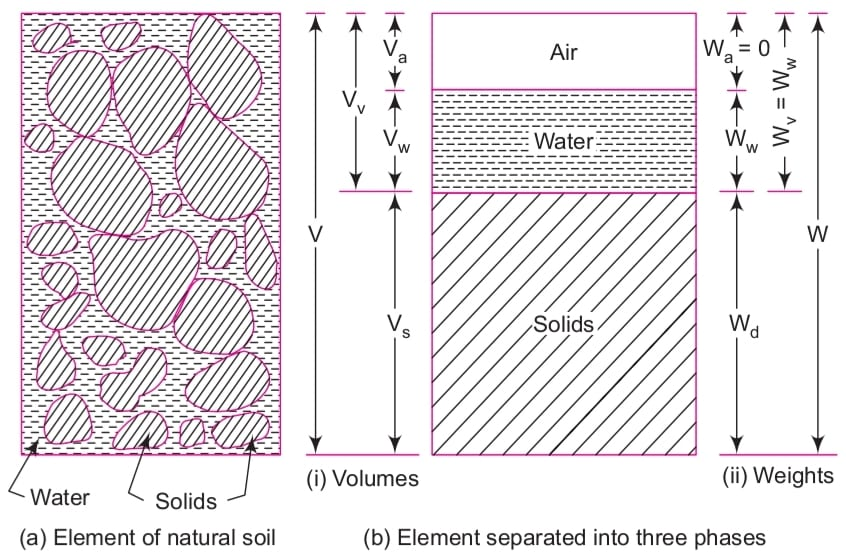### Soil Properties and Relation Between Them

Three-Phase System of Soil

• A Soil mass is a three-phase system consisting of solid particles (called soil grains) with water and air filling the void spaces between the soil grains. The void spaces are sometimes filled partly with air and water. But in the case of a dry soil mass, the voids are completely filled with air only and in the case of a saturated soil mass, the voids are completely filled with water.

• The properties of the soil mass which is a blend of the three constituents together forming a complex material depend on the relative percentages of these constituents, their arrangement and a variety of other factors.

Fig 2.1 Soil as a Three-Phase System• Terms And Symbols (Denoted By): Table-2.1

 V = Total Volume of the Soil Mass W = Total Weight of the Soil Mass Vs = Volume of Solids Wd = Weight of Solids Vv =Volume of Voids Wv = Weight of Voids Va = Volume of Air Wa = Weight of Air Vw = Volume of Water Ww = Weight of Water Note: a) V=Vs+Vv                   b) Vv=Va+Vw  In case of dry soil mass:    Vv=Va as Vw=0 In case of saturated soil mass: Vv=Vw as Va=0 Note: a) W=Wd+Wv b) Wv=Ww  as  Wa=0
• Table-2.2

 M = Total Soil Mass W = Total Weight of Soil Mass Mw = Mass of Water Ww = Weight of water Md = Mass Soil Solids Wd = Weight of Soil Solids (Md)sub = Mass of Submerged Soil Solids (Wd)sub= Weight of Submerged Soil Solids ρw = Density of Water ( 1000 kg/m3 ) γw = Unit Weight of Water ( 9.81 kN/m3 ) Inter-conversion between ρ and γ : ⏎ 1 γ (kN/m3)  = 9.81 ρ (g/cm3) γd,max = Maximum dry density from compaction test g = 9.81 m/s2 emax = Voids ratio, in the loosest state. 1N = 0.10197 kg emin = Voids ratio, in the densest state. 1 kg = 9.81 N e = Natural voice ratio of the deposit. 1m = 100cm = 1000mm